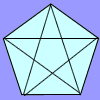#### You may also like### Number Detective

Follow the clues to find the mystery number.### Prime Magic

Place the numbers 1, 2, 3,..., 9 one on each square of a 3 by 3 grid so that all the rows and columns add up to a prime number. How many different solutions can you find?### Diagonal Trace

You can trace over all of the diagonals of a pentagon without lifting your pencil and without going over any more than once. Can the same thing be done with a hexagon or with a heptagon?

# Various Venns

##### Age 7 to 11 Challenge Level:

Where would you put a number which is square but not odd?
Where would a square odd number go?
Where would a number which is odd but not square go?
Where would you put a number which is not square and not odd?
You could try placing each number in turn. For example, is 0 a square number? Is it an odd number?